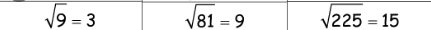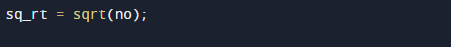C Program to Find Square Root of a Given Number

In this tutorial you will learn about the C Program to Find Square Root of a Given Number and its application with practical example.

In this tutorial, we will learn to create a C program that will find Square Root of given number  using C programming.

Prerequisites

Before starting with this tutorial we assume that you are best aware of the following C programming topics:

• C Operators.
• Basic input/output.
• In-built functions.
• mathematical functions.

What Is Square root?

Squares are found when numbers multiplying by itself 5 * 5= 25. Where a square root of a number on getting multiplied by itself gives the original √25 = 5. In other word a value that can be multiplied by itself to give the original number.C Program to Find Square Root of a Given Number.

Here in this program we are going find square root of a given number first of all we take a value from user and pass this value to in-built function to find square root of a number.

OutputIn the above program, we have first declared and initialized a set variables required in the program.

• no = Which will hold given value by user
• ctr = It will hols square root of a number.

Function sqrt() takes only a single parameter(no) and returns the square root .which we hold in sq_rt variable.with the help of sq_rt variable we can print the square of given number by user.Explanation

Explanation of Square root

Square root of a number is a value,that will occurs on multiplication by itself gives the original number. Any positive number, when multiplied by itself  5 * 5= 25 , represents the square of that number. The square root of the square of a positive number gives the original number √25 = 5.

Example

Input
Enter any number:  16
Output
Square root of 16 = 4.

In this tutorial we have learn about the C Program to Find Square Root of a Given Number and its application with practical example. I hope you will like this tutorial.